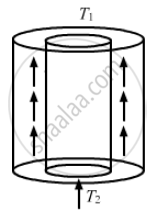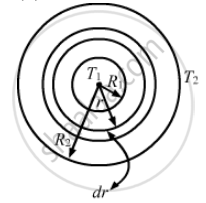Share

# A Hollow Tube Has a Length L, Inner Radius R1 and Outer Radius R2. the Material Has a Thermal Conductivity K. Find the Heat Flowing Through the Walls of the Tube If (A) the Flat Ends Are Maintained at - Physics

ConceptThermal Expansion of Solids

#### Question

A hollow tube has a length l, inner radius R1 and outer radius R2. The material has a thermal conductivity K. Find the heat flowing through the walls of the tube if (a) the flat ends are maintained at temperature T1 and T2 (T2 > T1) (b) the inside of the tube is maintained at temperature T1 and the outside is maintained at T2.

#### Solution

(a)  When the flat ends are maintained at temperatures T1 and T2 (where T2 > T1):

Area of cross section through which heat is flowing, = A = pi (R_2^2 - R_1^2)Rate of flow of heat = (d theta)/dt

= (KA ( Rpi - R_1^2) (T_2 - T_1))/l

( b )When the inside of the tube is maintained at temperature T1 and the outside is maintained at T2:

Let us consider a cylindrical shell of radius r and thickness dr.
Rate of flow of heat, q= KA. {aT}/{dr}

q = KA. (dt)/(dr)
q = K (2pirl)dt/(dr)
$\int\limits_{R1}^{R2}$ (dr)/r = 2piKl  $\int\limits_{T1}^{T2}$  dT
[In  (r)]_{R1 }^{R2}   (dr)/(r) =( 2pirL)/q  [T_2 - T_1]

In ((R_2)/(R_1)) = "2piKl"/ q [T_2 - T_1]

q = (2piKl(T_2-T_1))/"in" (R_2/R^1)`

Is there an error in this question or solution?

#### APPEARS IN

Solution A Hollow Tube Has a Length L, Inner Radius R1 and Outer Radius R2. the Material Has a Thermal Conductivity K. Find the Heat Flowing Through the Walls of the Tube If (A) the Flat Ends Are Maintained at Concept: Thermal Expansion of Solids.
S